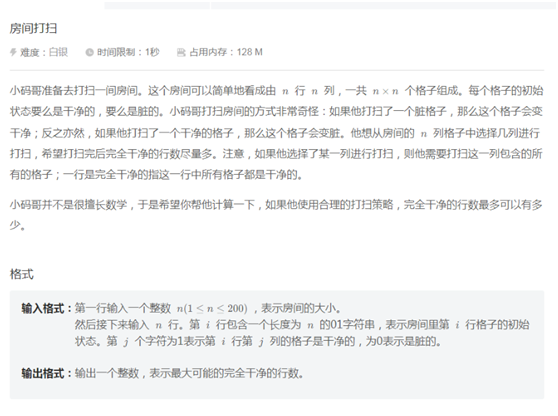SilyaSophie 2022-09-30 11:53 采纳率: 71.4%浏览 473

# 房间打扫C语言编程问题

###### 问题遇到的现象和发生背景###### 用代码块功能插入代码，请勿粘贴截图

#include<stdio.h>
void bubble_sort(int* p, int len)//函数实现
{
int i = 0;
int j = 0;
for (i = 0; i < len - 1; i++)//需要进行len-1趟
{
int flag = 1;//flag=1，说明已经排好序
for (j = 0; j < len - 1 - i;j++)//每趟两两比较较未排好序元素个数-1次。
{
if (p[j] < p[j+1])
{
int tmp = p[j];
p[j] = p[j + 1];
p[j + 1] = tmp;
flag = 0;
}
}
if (flag==1)//判断是否排好序
break;
}
}

int main()
{
int n,i,j,x,y=0;
scanf(“%d”,&n);
int a[n][n],b[n]=0;
for(i=0;i<n;i++)
{
for(j=0;j<n;j++)
{
scanf(“%d”,a[i][j]);
if(a[i][j]==0)
b[i]++;
}
}
scanf(“%d”,&x);
bubble_sort(b,n);
for (i = 0; i < x; i++)
{
y=y+b[x];
}
printf("%d\n",y/5);
return 0;
}
}

10011
01100
10100
01010
00011

• 写回答

#### 1条回答默认 最新

•[Pokemon]大猫猫 2022-09-30 15:57
关注

题主:你需要注意，输入的n行是字符串
提供这样一个思路，后续补充代码
比较n个字符串中相等字符串的最大数目，即为完全干净的最大行数

``````#include <stdio.h>
#include <string.h>

int main()
{

int n;
scanf("%d", &n);

int i, j;
char arr[n][n + 1]; //需要存储 '\0' 所以列数为 n + 1
for (i = 0; i < n; i++)
{
//arr[i] 表示第 i 行的数组名，即第 i 行的起始地址
scanf("%s", arr[i]);
}

int count = 0;
int ret = 0;
for (i = 0; i < n; i++)
{
count = 1;
for (j = i + 1; j < n; j++)
{
//arr[i] 表示第 i 行的字符串起始地址
if (strcmp(arr[i], arr[j]) == 0)
count++;
}

if (count > ret)
ret = count;
}

printf("%d\n", ret);
return 0;
}

``````

由于水平有限，只能做出如下改进：

``````#include <stdio.h>
#include <string.h>

int main()
{

int n;
scanf("%d", &n);

int i, j;
char arr[n][n + 1]; //需要存储 '\0' 所以列数为 n + 1
for (i = 0; i < n; i++)
{
//arr[i] 表示第 i 行的数组名，即第 i 行的起始地址
scanf("%s", arr[i]);
}

//标记数组，memset 将 tmp 数组初始化为全0
int tmp[n];
memset(tmp, 0, n * sizeof(int));

int count = 0;
int ret = 0;
for (i = 0; i < n; i++)
{
//该字符串已经确定出现的次数
if (tmp[i] != 0)
continue;

//已经可以确定出现的最大次数
if (ret >= n - i)
break;

count = 1;
for (j = i + 1; j < n; j++)
{
//arr[i] 表示第 i 行的字符串起始地址
//未确定出现次数的字符串才进行比较
if (tmp[j] == 0 && strcmp(arr[i], arr[j]) == 0)
{
count++;
tmp[j] = 1;    //标记该字符串已经确定了出现的次数
}
}

if (count > ret)
ret = count;
}

printf("%d\n", ret);
return 0;
}

``````
本回答被题主选为最佳回答 , 对您是否有帮助呢?
评论 编辑记录

#### 悬赏问题

• ¥15 C++代码报错问题，c++20协程
• ¥15 c++图Djikstra算法求最短路径
• ¥15 Linux操作系统中的，管道通信问题
• ¥15 ansible tower 卡住
• ¥15 等间距平面螺旋天线方程式
• ¥15 通过链接访问，显示514或不是私密连接
• ¥100 系统自动弹窗，键盘一接上就会
• ¥50 股票交易系统设计（sql语言）
• ¥15 调制识别中这几个数据集的文献分别是什么？
• ¥15 使用c语言对日志文件处理